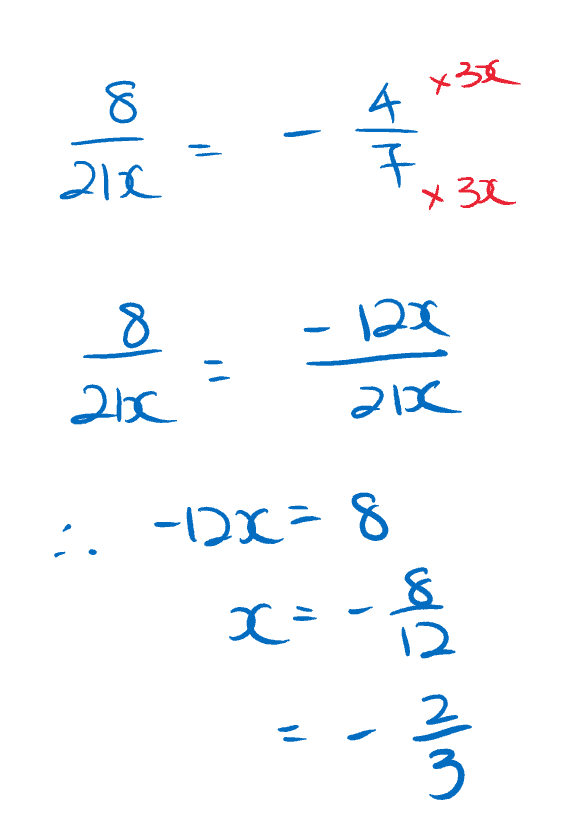# Question0 Replies 1 Like ✔Accepted Answer

Multiplying 7 to both sides, we get:

8*7 / 21x = -4*7/7 = -4

Multiplying 3x to both sides, we get

8 * 3x / 3x = -4 * 3x = -12x

8 = – 12 x

Hence, x = -8/12 = -2/3

0 Replies 0 Likes